# Chapter 10 Example

## Example Exam Questions

Question 1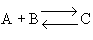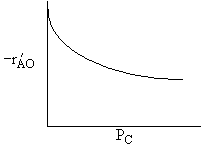Figure 1: Data from a differential reactor. Which of the following best describe the data in the above figure? Species C could be adsorbed on the surface. The reaction could be reversible. Both the above statements could be true. Neither A or B are on the surface. None of the above could be true.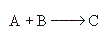Assume the reaction is irreversible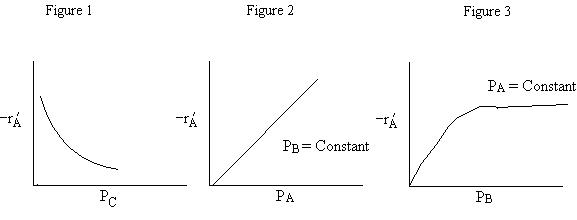What can you tell from the above figures? Species A could be adsorbed on the surface but only very weakly adsorbed. Species A is not adsorbed on the surface. Species B is on the surface. Species A is adsorbed on the surface. Species C is adsorbed on the surface. 1 and 2 are true. 1 and 3 are true. 1 and 4 are false. 4 and 5 are true. 2 and 5 are true.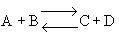The following data were reported for the reaction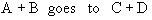A B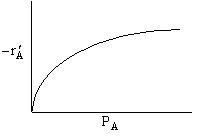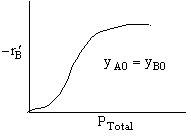C D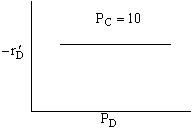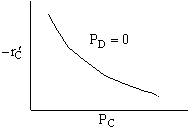Which of the following mechanism is consistent with the above data

 (a) (b) (c)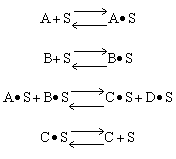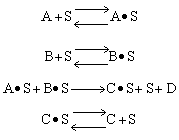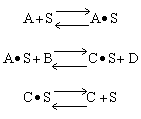(d) (e)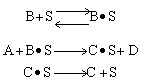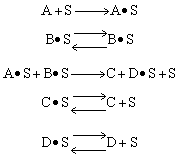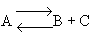The initial rate of reaction is shown below

 (a) (b) (c)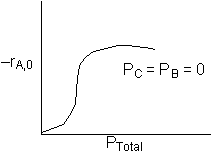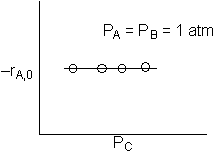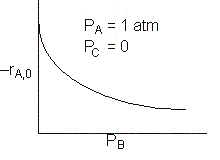The reaction is irreversible. Species B is on the surface. Species C is on the surface. 1 and 2 are true. 1 and 2 are false. 1 and 3 are false. 2 and 3 are false. 2 and 3 are true.The initial rate of reaction is shown below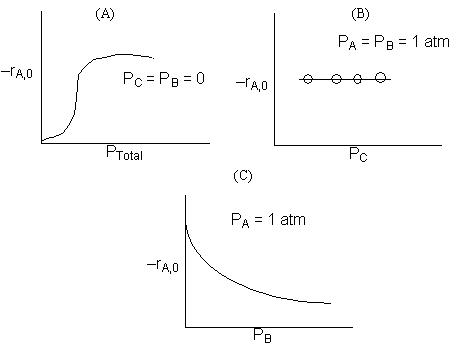1. The rate law is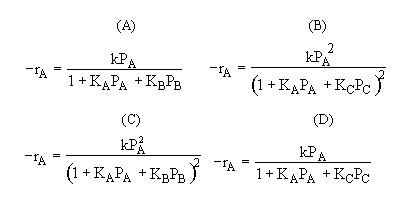For the reactionfrom Self Tests 12 and 13 we found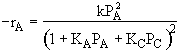which mechanism is consistent with the rate law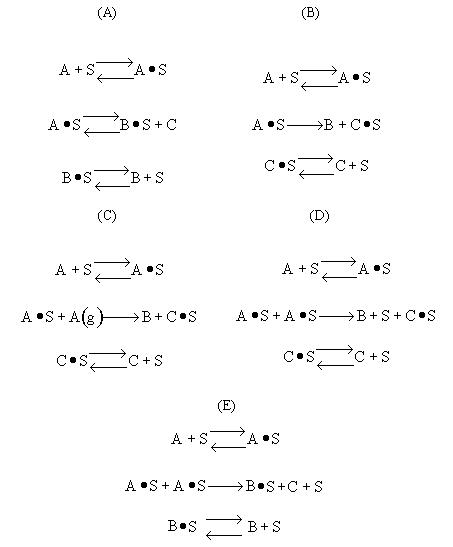If the reaction is reversible the increase in PC will decrease the rate. If C is on the surface it will be in the denomination of the rate law and thus increasing PC will decrease the rate.   Ans: C

Problem #2

 A is not on the surface or if it is KAPA << 1.  B is on the surface and we see as we increase PB, the rate reaches a plateau. The curves from A, B, and C partial pressure suggest a rate law of the for Figure 1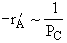Figure 2Figure 3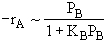Combining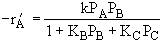Possible Mechanism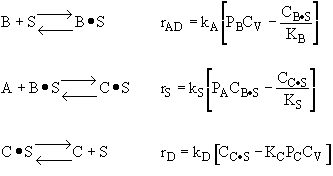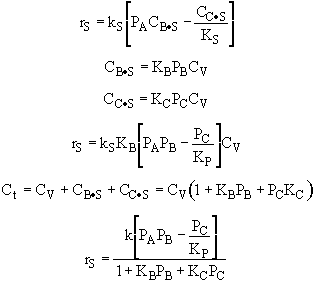From the rate law we know species B and C are adsorbed on the surface. From the data you can’t tell whether or not C is adsorbed on the surface. Ans: E

Problem #3Recall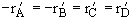Figure (a) suggests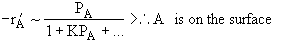Figure (b) suggests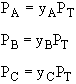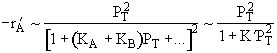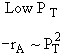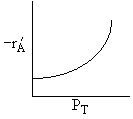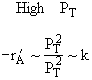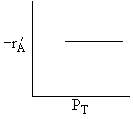Combining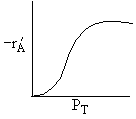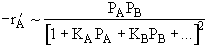Figure (c) suggests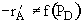therefore reaction is irreversible and D is not on surface

Figure (d) suggests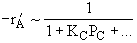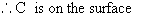Combining all the above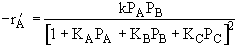Therefore (b) is consistent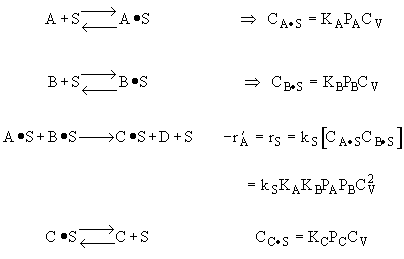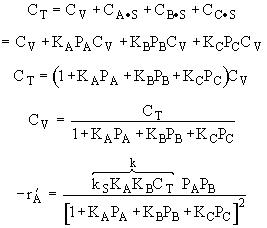Problem 4

 1.   The reaction is irreversible because when PB = 1 atm, increasing the product C does not change the rate. (TRUE) 2.   Species B is on the surface because in 1 we showed that the reaction is irreversible, therefore the only way the rate could decrease were if were in the denominator of the rate law. If a species is in the denominator of the rate law it’s on the surface. (TRUE) 3.   Species C is not on the surface because increasing PC does not affect the rate.(FALSE) Ans: A

Problem #5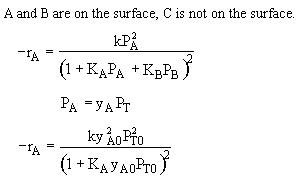Low PT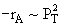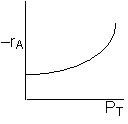High PT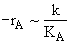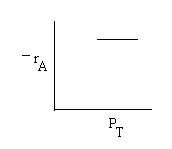Combining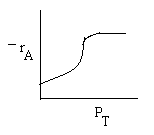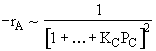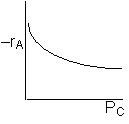Ans: (c)

Problem #6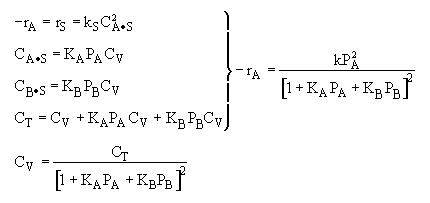Ans: (e)

Back to Chapter 10